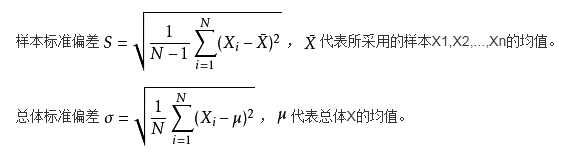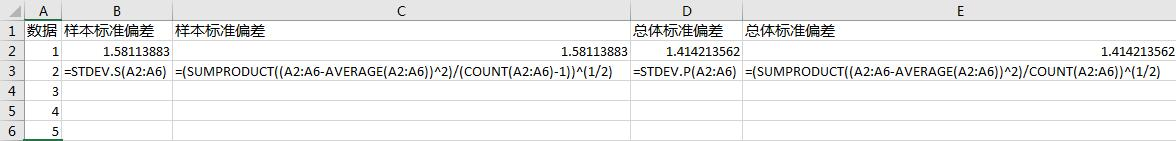﻿ 如何用excel或wps计算标准差、方差、标准误差？ - Excel - ExcelOffice【微信公众号：水星Excel】

# 如何用excel或wps计算标准差、方差、标准误差？

## 一、标准差的计算```'样本标准偏差
=STDEV.S(A2:A6)
'样本标准偏差
=(SUMPRODUCT((A2:A6-AVERAGE(A2:A6))^2)/(COUNT(A2:A6)-1))^(1/2)
'总体标准偏差
=STDEV.P(A2:A6)
'总体标准偏差
=(SUMPRODUCT((A2:A6-AVERAGE(A2:A6))^2)/COUNT(A2:A6))^(1/2)
```

## 三、标准误差的计算

‘标准误差

=STDEV.S(A2:A6)/SQRT(COUNT(A2:A6))